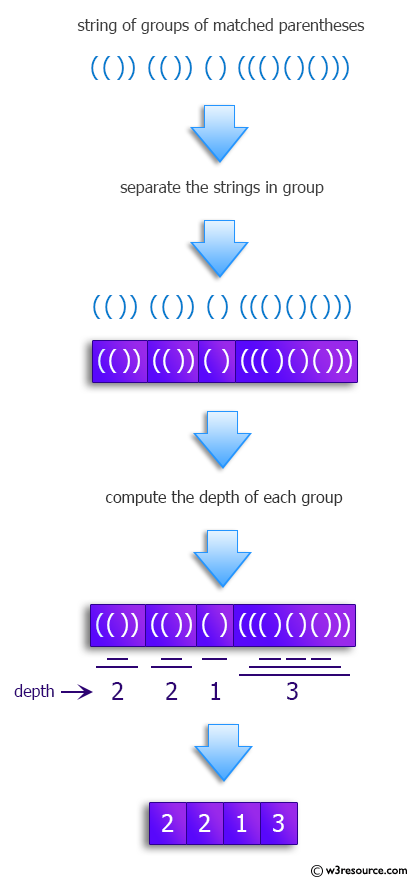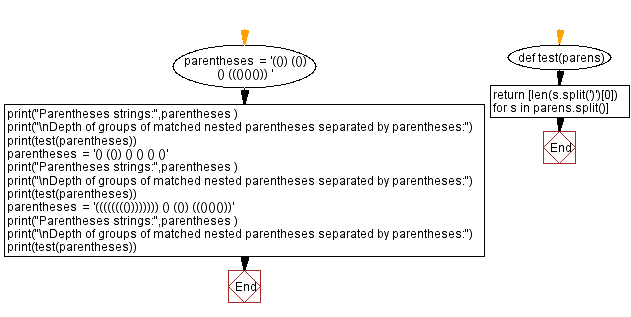﻿ Python: Compute the depth of groups of matched nested parentheses separated by parentheses - w3resource# Python: Compute the depth of groups of matched nested parentheses separated by parentheses

## Python Programming Puzzles: Exercise-98 with Solution

Given a string consisting of groups of matched nested parentheses separated by parentheses, write a Python program to compute the depth of each group.

```Input: (()) (()) () ((()()()))

Output:
[2, 2, 1, 3]
Input: () (()) () () () ()

Output:
[1, 2, 1, 1, 1, 1]
Input: (((((((()))))))) () (()) ((()()()))

Output:
[8, 1, 2, 3]
```

Pictorial Presentation:Sample Solution:

Python Code:

``````#License: https://bit.ly/3oLErEI

def test(parens):
return [len(s.split(')')) for s in parens.split()]

parentheses  = '(()) (()) () ((()()())) '
print("Parentheses strings:",parentheses )
print("\nDepth of groups of matched nested parentheses separated by parentheses:")
print(test(parentheses))
parentheses  = '() (()) () () () ()'
print("Parentheses strings:",parentheses )
print("\nDepth of groups of matched nested parentheses separated by parentheses:")
print(test(parentheses))
parentheses  = '(((((((()))))))) () (()) ((()()()))'
print("Parentheses strings:",parentheses )
print("\nDepth of groups of matched nested parentheses separated by parentheses:")
print(test(parentheses))
``````

Sample Output:

```Parentheses strings: (()) (()) () ((()()()))

Depth of groups of matched nested parentheses separated by parentheses:
[2, 2, 1, 3]
Parentheses strings: () (()) () () () ()

Depth of groups of matched nested parentheses separated by parentheses:
[1, 2, 1, 1, 1, 1]
Parentheses strings: (((((((()))))))) () (()) ((()()()))

Depth of groups of matched nested parentheses separated by parentheses:
[8, 1, 2, 3]
```

Flowchart:## Visualize Python code execution:

The following tool visualize what the computer is doing step-by-step as it executes the said program:

Python Code Editor :

Have another way to solve this solution? Contribute your code (and comments) through Disqus.

Previous: Strange sort of list of numbers.
Next: Expand Spaces.

What is the difficulty level of this exercise?

Test your Programming skills with w3resource's quiz.

﻿

## Python: Tips of the Day

Clamps num within the inclusive range specified by the boundary values x and y:

Example:

```def tips_clamp_num(num,x,y):
return max(min(num, max(x, y)), min(x, y))
print(tips_clamp_num(2, 4, 6))
print(tips_clamp_num(1, -1, -6))
```

Output:

```4
-1
```